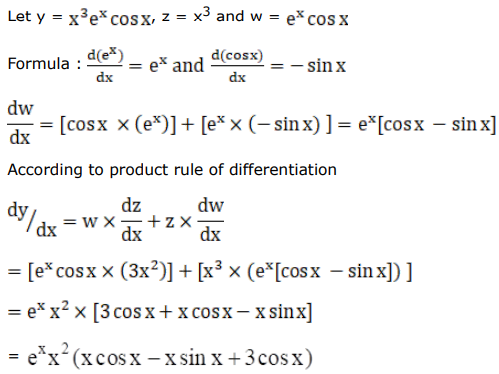# Solve this followingQuestion:

Differentiate each of the following w.r.t. $x$ :

$x^{3} e^{x} \cos x$

Solution: